# Difference between revisions of "Activities/Turtle Art/Numbers"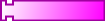number used as numeric input in mathematic operators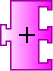addition adds two numeric inputssubtraction subtracts bottom numeric input from top numeric input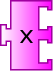product multiplies two numeric inputs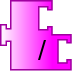division divides top numeric input (numerator) by bottom numeric input (denominator)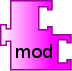mod modular (remainder) operator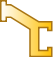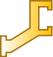numeric extensions identity operators used for extending blocks (See example above)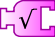square root calculate square root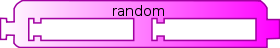random returns random number between minimum (left) and maximum (right) valuesequal? logical = operator (plugs into an If blocks on the Flow Palette)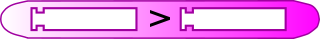greater? logical > operator (plugs into an If blocks on the Flow Palette)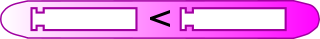less? logical < operator (plugs into an If blocks on the Flow Palette)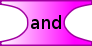and logical ⋀ (and) operator (links conditional)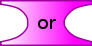or logical ⋁ (or) operator (links conditional)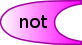not logical ￢ (not) operator (links conditional)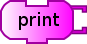print Prints value in status block at bottom of the canvas (See example above)